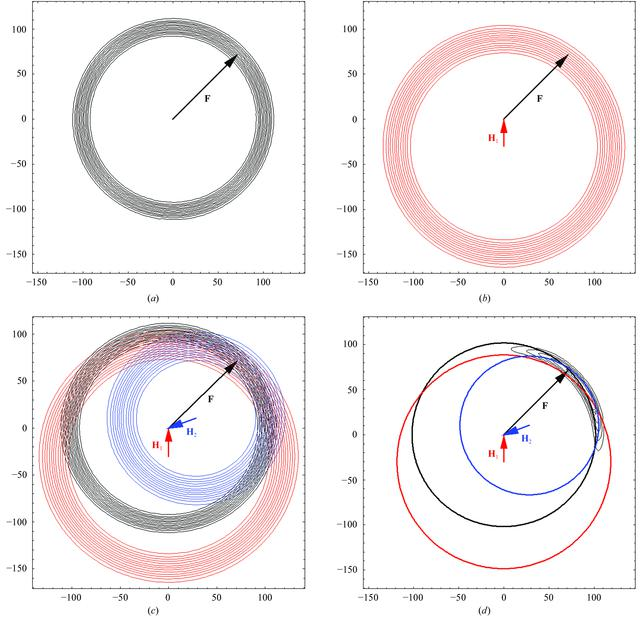disable zoom     view article Figure 2 Schematic representation of the interpretation of (7)as a probabilistic version of the Harker (1956) construction. Each panel shows a contour plot of a probability distribution. (a) shows the probability distribution for the observed native amplitude as a function of possible values for the true native structure factor. The width of this distribution represents the estimated error in measurement of the native amplitude. (b) shows the probability distribution for the observed amplitude for derivative 1, again as a function of possible values for the true native structure factor. Because the derivative structure factor must be the sum of the heavy-atom contribution and the protein contribution, the centre of the circularly symmetric distribution is offset by minus the heavy-atom contribution. (c) overlays the first two distributions, as well as the distribution for a second derivative, and (d) shows the product of all three distributions as a function of the assumed true native structure factor. The combined distribution has significant values where the circles from the Harker construction come close to intersecting.BIOLOGICALCRYSTALLOGRAPHY
ISSN: 1399-0047
Volume 59| Part 11| October 2003| Pages 1891-1902